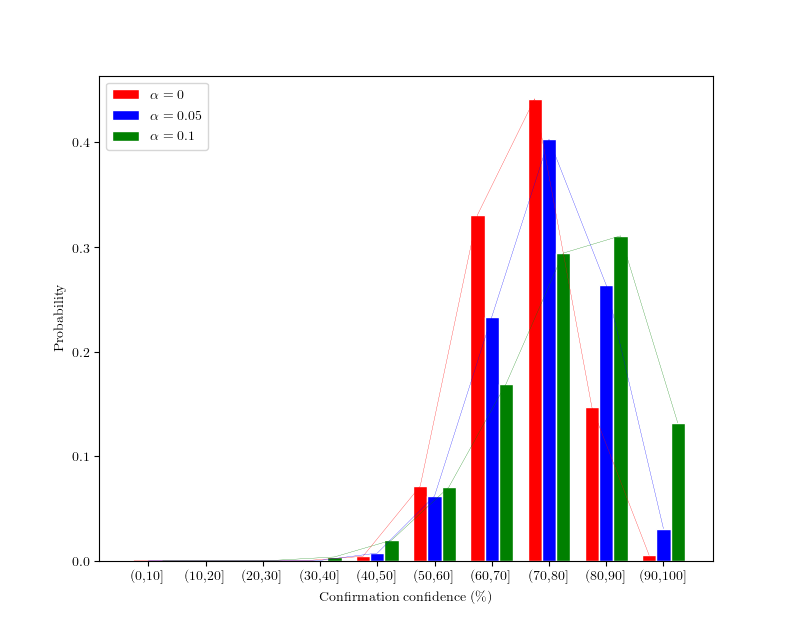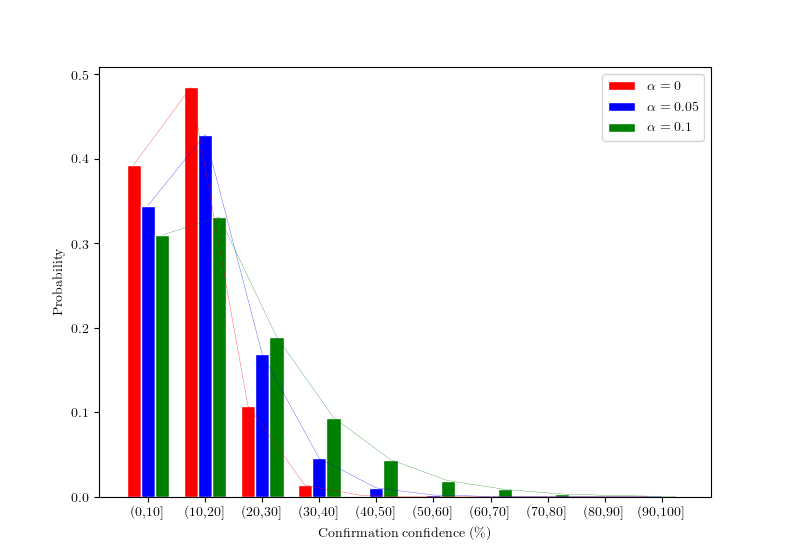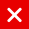# Confirmation and ɑ

Currently, we do not need any measure of acceptance of a transaction, since the coordinator issues milestones, which provides us a simple rule to decide whether a transaction should or should not be accepted. But what would happen after the coordicide (what we are calling the death of the coordinator)? For this purpose, the confirmation confidence (which will be called CC hereafter) was defined in the Whitepaper as a post-coordicide metric of a transaction’s acceptance by the nodes. In this post, we will present how this metric’s behaviour depend on the parameter ɑ of the tip selection algorithm.

The CC of a certain transaction is obtained by releasing a large number of random walks and counting how many of these walks end on a tip that references the transaction. The fraction of tips which references it will be its CC. So, if a certain transaction has a CC close to 1, the recipient of the funds should be confident that he will be able to spend them later. Additionally, if the user knows how the CC of a normal, healthy transaction looks like, he might infer if his transaction is maturing normally. To illustrate this, let us consider a hypothetical situation in this post-coordicide scenario, where our default measure of acceptance of a transaction is the CC. You own a bookstore and a customer wants to buy a book paying in IOTAs. Two seconds after the payment the CC of the transaction is 1%. Does it mean that the buyer is trying to trick you? The answer is quite intuitive for anyone that has already used cryptocurrencies: No, since the transaction is not mature enough to get to any conclusion. However, in a healthy Tangle, the CC should increase fast. Now, suppose that, the next morning, the transaction’s CC it is still at 10%. In this case, you should start worrying, because the transaction is too old to have such a low CC. So it is clear that the CC of a transaction is a dynamic property which must be analyzed taking into account the transaction’s age.

Now, suppose that a transaction has a CC = 45% at a certain time. In the absence of adversities, what should we expect to be the CC after, let us say, 2 seconds? We plotted some graphs to try to answer this question. For that, we examined the evolution of 80k transactions for three values of ɑ and a fixed transaction arrival rate λ=25. We study the future evolution of the CC in two meaningful scenarios that correspond to transactions showing a medium acceptance (set A) and a low acceptance (set B):

Set A — transactions that, at time t, presented a CC in the range (40%, 50%), meaning that they had a medium acceptance.

Set B — transactions that, at time t, presented a CC in the range (0%, 10%), meaning that they had a low acceptance.

We answer the question: If a transaction has a given CC at time t, what do we expect to be its CC at time t+2s?

Figure 1 shows the CC probability distribution at time t+2s, for set A. We remind that these transactions have a CC of between 40% and 50% at time t. Each color corresponds to one value of ɑ. The y axis stands for the probability of the CC being in each interval in the x axis. For example, the larger red bar in the graph means that a transaction of this type should have a ~50% (the amount in the y axis) of chance of being in the interval (70%,80%) (the interval in the x axis) at time t+2s, when ɑ = 0 (corresponding at the color of the bar).Figure 1 — Probability distribution of the CC at time t+2s, for transactions that had a CC in the interval (40%,50%) at time t.

Now notice how a higher ɑ accelerates this CC evolution. The green bars in the first figure are visibly larger than the others in the higher intervals and smaller in the lower ones. The peak of the distribution is also shifted to the right with an ɑ increase.

Figure 2 is similar to the first one, but now we look at the set B transactions. Here, the probability that the CC remains at a very low value is higher for the larger ɑ. At the same time, the probability of the CC reaching high values grows with ɑ, indicating that the distribution is somehow wider. The explanation of this behaviour, apparently contradictory, lies in the fact that, for ɑ>0, we expect that some tips will be left behind. So, what happens if we exclude these tips that are left behind? This situation is depicted in Figure 3.Figure 2- Probability distribution of the CC at time t+2s, given that the CC was in the interval (0%,10%) at time t.Figure 3 — Probability distribution of the CC at time t+2s, given that the CC was in the interval (0%,10%) at time t, excluding tips left behind.

Comparing Figure 2 and 3, it is clear the presence of two opposite forces: on the one hand, the CC will quickly increase if ɑ is high; on the other hand, a large ɑ increases the number of tips that are left behind. This can be explained by the fact that, the larger the value of ɑ, the more deterministic our tip selection algorithm is, meaning that a larger ɑ will force the algorithm to prefer popular transactions over unpopular ones.

In this post, we qualitatively described how the CC evolution is affected by the parameter ɑ, for a fixed transactions arrival rate λ and in the absence of adversities. However, would this CC evolution be any different in the presence of a double spending? What about multiple spendings? Is the average CC always increasing for every range of parameters? These, among other questions, are still being investigated, but their answers are of great importance for a complete understanding of the system’s behavior.

You might also like

This website uses cookies to improve your experience. We'll assume you're ok with this, but you can opt-out if you wish. AcceptRead More

Did you know?

Buy in without the stress of trying to time the market

when you setup a savings plan. If you haven’t started a plan yet, head to your Bitpanda account to get started!

Purchasing cryptocurrencies comes with a number of risks.
Never invest money that you can't afford to lose.You can automatically invest

in IOTA with Bitpanda Savings!